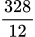Price Comparison between Appsero and Other Platforms
Share
Explore

#Appsero+FastSpring vs WooCommerce+Stripe

💰 Appsero+FastSpring Cost
Appsero + FastSpring Cost Calculation
18
Search
Product Price
Appsero Fee
FastSpring 95C
FastSpring 5.9%
Total FastSpring Cost
FastSpring + Appsero Total Cost
1
50
$25.00$20.00
47.5
$73.75$121.25
$141.25 2 250$25.00
$20.00 237.5$368.75
$606.25$626.25
3
500
$25.00$20.00
475
$737.50$1,212.50
$1,232.50 4 1000$25.00
$57.50 950$1,475.00
$2,425.00$2,482.50
5
1500
$25.00$57.50
1425
$2,212.50$3,637.50
$3,695.00 6 2500$25.00
$82.50 2375$3,687.50
$6,062.50$6,145.00
7
3500
$25.00$82.50
3325
$5,162.50$8,487.50
$8,570.00 8 5000$25.00
$157.50 4750$7,375.00
$12,125.00$12,282.50
9
7500
$25.00$157.50
7125
$11,062.50$18,187.50
$18,345.00 10 10000$25.00
$290.83 9500$14,750.00
$24,250.00$24,540.83
There are no rows in this table
💰 Cost for WooCommerce (Using WooCommerce Subscription, Software Add-on & Stripe Payment Gateway)
View of WooCommerce Plugins Cost
1
Search
Plugin Name
Annual Cost
1
$129.00 2 WooCommerce Subscriptions$199.00
There are no rows in this table
$328.00 Sum Monthly Cost WooCommerce Fee=$27.33
Cost for WooCommerce with Plugins 2
18
Search
Product Price
WooCommerce Fee
Stripe 3.4%
Stripe 50C
Total Cost with WooCommerce + Stripe
FastSpring + Appsero Total Cost
1
50
$25.00$27.33
$42.50 25$94.83
$141.25 2 250$25.00
$27.33$212.50
125
$364.83$626.25
3
500
$25.00$27.33
$425.00 250$702.33
$1,232.50 4 1000$25.00
$27.33$850.00
500
$1,377.33$2,482.50
5
1500
$25.00$27.33
$1,275.00 750$2,052.33
$3,695.00 6 2500$25.00
$27.33$2,125.00
1250
$3,402.33$6,145.00
7
3500
$25.00$27.33
$2,975.00 1750$4,752.33
$8,570.00 8 5000$25.00
$27.33$4,250.00
2500
$6,777.33$12,282.50
9
7500
$25.00$27.33
$6,375.00 3750$10,152.33
$18,345.00 10 10000$25.00
$27.33$8,500.00
5000
$13,527.33$24,540.83
There are no rows in this table
💰 Total Cost Percentage Comparing Revenue
Total Cost Percentage When You Use WooCommerce+Stripe 🆚 Appsero+FastSpring
18
Search
Product Price
Total Revenue
% Cost Appsero with FastSpring
Percent Cost WooCommerce with Stripe
1
50
$25.00$1,250.00
11.3%
7.59%
2
250
$25.00$6,250.00
10.02%
5.84%
3
500
$25.00$12,500.00
9.86%
5.62%
4
1000
$25.00$25,000.00
9.93%
5.51%
5
1500
$25.00$37,500.00
9.85%
5.47%
6
2500
$25.00$62,500.00
9.83%
5.44%
7
3500
$25.00$87,500.00
9.79%
5.43%
8
5000
$25.00$125,000.00
9.83%
5.42%
9
7500
$25.00$187,500.00
9.78%
5.41%
10
10000
$25.00$250,000.00
9.82%
5.41%
There are no rows in this table
Graph Comparison
How much do you spend in each of the platforms? 2
18